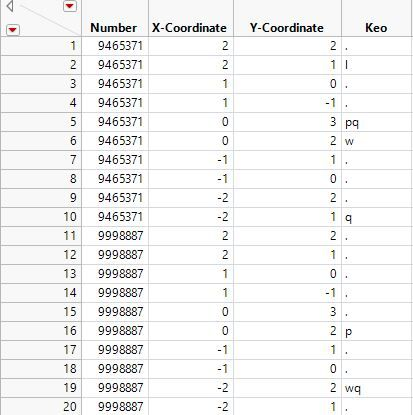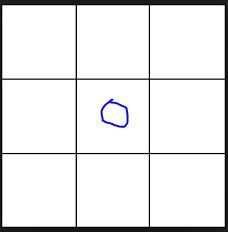Choose Language Hide Translation Bar
Highlighted

## Very Complicated Coordinates

Hi everyone.

I have this very complicated question to explain over here. So basically, this is the data table:I'm supposed to create a column called "Total Coordinates Around You"  using JSL script, by imagining that there are 8 squares around the particular coordinate (excluding its own coordinate basically), as shown by the picture below, where the small blue circle represents this particular coordinate. Thus, the maximum "Total Coordinates Around You" is 8. For example, as shown by the picture above, by the number 9998887, coordinate (-2,1) has got a "Total Coordinates Around You" of 3, which are (-2,2), (-1,1) and (-1,0). Just for a note, the horizontal axis represents shift in x-coordinate and likewise, the vertical axis represents shift in x-coordinate. From the data table above, it is easy to make 20 "Total Coordinates Around You", but in my actual tasks, there are about 23,000+ rows to be dealt with. Also, one more thing, I'm supposed to sort the numbers according, like 9465371 and 9998887 shown in the data table above, respectively (they got the same coordinates), before working on the "Total Coordinates Around You".``````dt = current data table();

dt << New column ("Total Coordinates Around You",
numeric,
continuous,
formula (
For ( i = 1, i<= N Row(), i++,
(:Total Coordinates Around You = 0; munipulated_x = :X-Coordinate - 1; munipulated_y = Y-Coordinate - 1; j = 1; k = 1; formula (
For ( j = 1, j <= 3, j++,
(formula(
For ( k = 1, k <= 3, k++,
(formula( If (munipulated_x == :X-Coordinate & munipulated_y == :Y-Coordinate, :Total Coordinates Around You))
; munipulated_y ++)
)
) ; munipulated_x ++)
)
) ; :Total Coordinates Around You --)
)
)
);``````

Above is a script which I created, which I'm not sure why this can't be worked, using loops. Can someone enlighten me on the steps and solutions on how to deal with this problem? There is a second part to this question, which I will try once I get my first part done. Do allow me to explain should there be any doubt especially on the clarity of the question and explanation, and once again, pardon me for the explanation. Thank you.

1 ACCEPTED SOLUTION

Accepted Solutions
Highlighted

## Re: Very Complicated Coordinates

I wouldn't do it in a formula because it runs for each row, so looping inside of a formula will cause you to be pretty slow.

This probably isn't the best way but it seems to work.  Assuming I understand correctly and you're trying to find how many tiles are touching your beginning tile?

Let me know if this is what you're trying to do.

``````Names default to here(1);
dt = New Table( "Example",
New Column( "Number",
Numeric,
"Continuous",
Format( "Best", 12 ),
Set Values(
[9465371, 9465371, 9465371, 9465371, 9465371, 9465371, 9465371, 9465371,
9465371, 9465371, 9998887, 9998887, 9998887, 9998887, 9998887, 9998887,
9998887, 9998887, 9998887, 9998887]
)
),
New Column( "X-Coordinate",
Numeric,
"Continuous",
Format( "Best", 12 ),
Set Values(
[2, 2, 1, 1, 0, 0, -1, -1, -2, -2, 2, 2, 1, 1, 0, 0, -1, -1, -2, -2]
)
),
New Column( "Y-Coordinate",
Numeric,
"Continuous",
Format( "Best", 12 ),
Set Selected,
Set Values( [2, 1, 0, -1, 3, 2, 1, 0, 2, 1, 2, 1, 0, -1, 3, 2, 1, 0, 2, 1] )
),
);

summarize(dt, byn = By(:Number));
for(i=1, i<=nitems(byn), i++,
sel = byn[i];
rows = dt << Get Rows Where(:Number == num(sel));
mat = dt[rows, {"X-Coordinate", "Y-Coordinate"}];
for(k = 1, k<=nrows(rows), k++,
row = rows[k];
// do a check that you're within the limit
adj = sqrt( (mat[k, 1] - mat[0, 1])^2 + (mat[k, 2] - mat[0, 2])^2) <= sqrt(2);

)
);``````

If you HAD to have it as a formula, this will get the same answer.

``````dt << New Column("Adjacent Formula", Formula(
dt = Current Data Table();
rows = Loc( As List( :Number << Get Values ), :Number );
mat = dt[rows, {"X-Coordinate", "Y-Coordinate"}];
Sum(
Sqrt(
(:Name( "X-Coordinate" ) - mat[0, 1]) ^ 2 + (:Name( "Y-Coordinate" ) - mat[0,
2]) ^ 2
) <= Sqrt( 2 )
) - 1;
))
``````

Vince Faller - Predictum
7 REPLIES 7
Highlighted

## Re: Very Complicated Coordinates

I wouldn't do it in a formula because it runs for each row, so looping inside of a formula will cause you to be pretty slow.

This probably isn't the best way but it seems to work.  Assuming I understand correctly and you're trying to find how many tiles are touching your beginning tile?

Let me know if this is what you're trying to do.

``````Names default to here(1);
dt = New Table( "Example",
New Column( "Number",
Numeric,
"Continuous",
Format( "Best", 12 ),
Set Values(
[9465371, 9465371, 9465371, 9465371, 9465371, 9465371, 9465371, 9465371,
9465371, 9465371, 9998887, 9998887, 9998887, 9998887, 9998887, 9998887,
9998887, 9998887, 9998887, 9998887]
)
),
New Column( "X-Coordinate",
Numeric,
"Continuous",
Format( "Best", 12 ),
Set Values(
[2, 2, 1, 1, 0, 0, -1, -1, -2, -2, 2, 2, 1, 1, 0, 0, -1, -1, -2, -2]
)
),
New Column( "Y-Coordinate",
Numeric,
"Continuous",
Format( "Best", 12 ),
Set Selected,
Set Values( [2, 1, 0, -1, 3, 2, 1, 0, 2, 1, 2, 1, 0, -1, 3, 2, 1, 0, 2, 1] )
),
);

summarize(dt, byn = By(:Number));
for(i=1, i<=nitems(byn), i++,
sel = byn[i];
rows = dt << Get Rows Where(:Number == num(sel));
mat = dt[rows, {"X-Coordinate", "Y-Coordinate"}];
for(k = 1, k<=nrows(rows), k++,
row = rows[k];
// do a check that you're within the limit
adj = sqrt( (mat[k, 1] - mat[0, 1])^2 + (mat[k, 2] - mat[0, 2])^2) <= sqrt(2);

)
);``````

If you HAD to have it as a formula, this will get the same answer.

``````dt << New Column("Adjacent Formula", Formula(
dt = Current Data Table();
rows = Loc( As List( :Number << Get Values ), :Number );
mat = dt[rows, {"X-Coordinate", "Y-Coordinate"}];
Sum(
Sqrt(
(:Name( "X-Coordinate" ) - mat[0, 1]) ^ 2 + (:Name( "Y-Coordinate" ) - mat[0,
2]) ^ 2
) <= Sqrt( 2 )
) - 1;
))
``````

Vince Faller - Predictum
Highlighted

## Re: Very Complicated Coordinates

Hi @vince_faller,

Thank you for your help. I'm actually trying to see if the corresponding coordinates of DieX and DieY exist, like what you're said, tiles touching the beginning tile, and so on and so forth, for 20 rows, so I'm not sure how to create a formula without having to use the loop like what you've mentioned. Also, your second part's formula:

Sum(
Sqrt(
(:Name( "X-Coordinate" ) - mat[0, 1]) ^ 2 + (:Name( "Y-Coordinate" ) - mat[0,
2]) ^ 2
) <= Sqrt( 2 )
) - 1;

only takes into the account of matrix [0,0], am I right to say that? Thank you.
Highlighted

## Re: Very Complicated Coordinates

Can you describe what you're actually trying to do instead then?
And no that isn't just for mat[0,0].
Vince Faller - Predictum
Highlighted

## Re: Very Complicated Coordinates

Hi @vince_faller,

I'm sorry, I think I'm getting there but can I just perhaps understand what this line of yours: adj = sqrt( (mat[k, 1] - mat[0, 1])^2 + (mat[k, 2] - mat[0, 2])^2) <= sqrt(2); mean? Thank you.
Highlighted

## Re: Very Complicated Coordinates

Is the issue that you don't think a For() loop can be used in a formula? It certainly can be used in a formula
Jim
Highlighted

## Re: Very Complicated Coordinates

I'm not sure I understand the requirement, but if you want to find neighbouring points to a given point, you could also onsider using 'KDTable()'. For example:

``````Names Default To Here( 1 );

n = 100;		// Size of square

// Make a table of x and y locations
dtx = AsTable((1::n)`, << Invisible);
dty = AsTable((1::n)`, << Invisible);
dt = dty << Join( With( dtx ), Cartesian Join );
Close(dtx, NoSave);
Close(dty, NoSave);
Column(dt, 1) << setName("x");
Column(dt, 2) << setName("y");
dt << deleteProperty("Source");
dt << setName("xy Locations");

// Put thes locations into a matrix
mat = dt << getAsMatrix;
tbl = KDTable( mat );

// Pick a point in the interior, so we don't need to decide on any boundary conditions
myX = RandomInteger(2, n-1);
myY = RandomInteger(2, n-1);
myRow = Loc(mat[0, 1] == myX & mat[0, 2] == myY);
myRow = myRow;

// Find the 8 nearest neighbours to the chosen point
{rows, dist} = tbl << K nearest rows( 8, myRow );
Show( myRow, rows );

// Check it worked
myXvals = Column(dt, "x")[myRow];
myYvals = Column(dt, "y")[myRow];
dt << NewColumn("Distance", Numeric, Continuous, Formula(sqrt((:x - myXvals)^2 +(:y - myYvals)^2)));
dt << selectRows(VConcat(myRow, rows`));
gb = dt << Graph Builder(
Size( 529, 453 ),
Show Control Panel( 0 ),
Variables( X( :x ), Y( :y ), Color( :Distance ) ),
Elements( Points( X, Y, Legend( 5 ) ) )
);
``````
Highlighted

## Re: Very Complicated Coordinates

Hi everyone @vince_faller, @txnelson and @ian_jmp, I've got the question requirement already, sorry for the unclear question instruction and confusion made, thank you! :)
Article Labels

There are no labels assigned to this post.